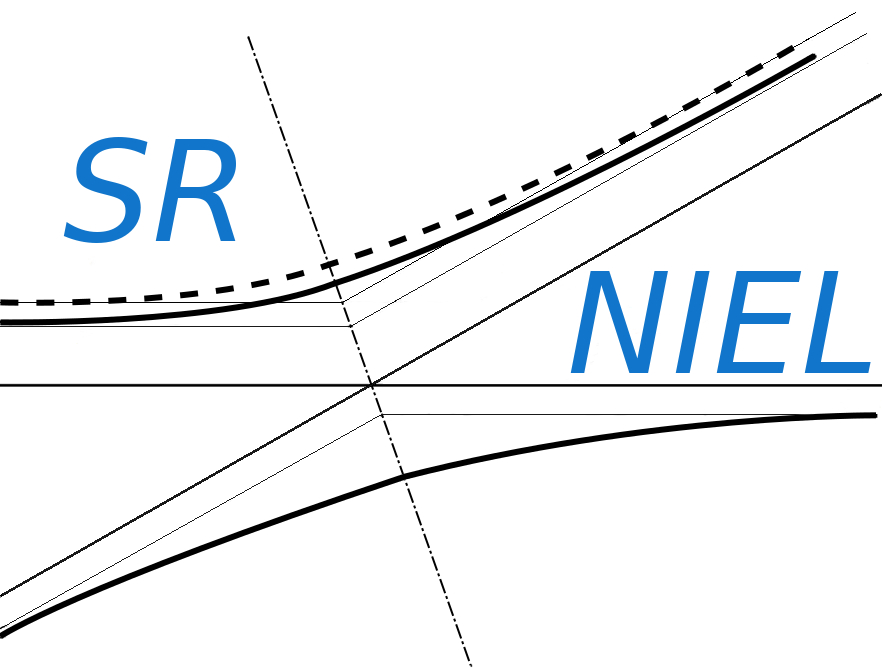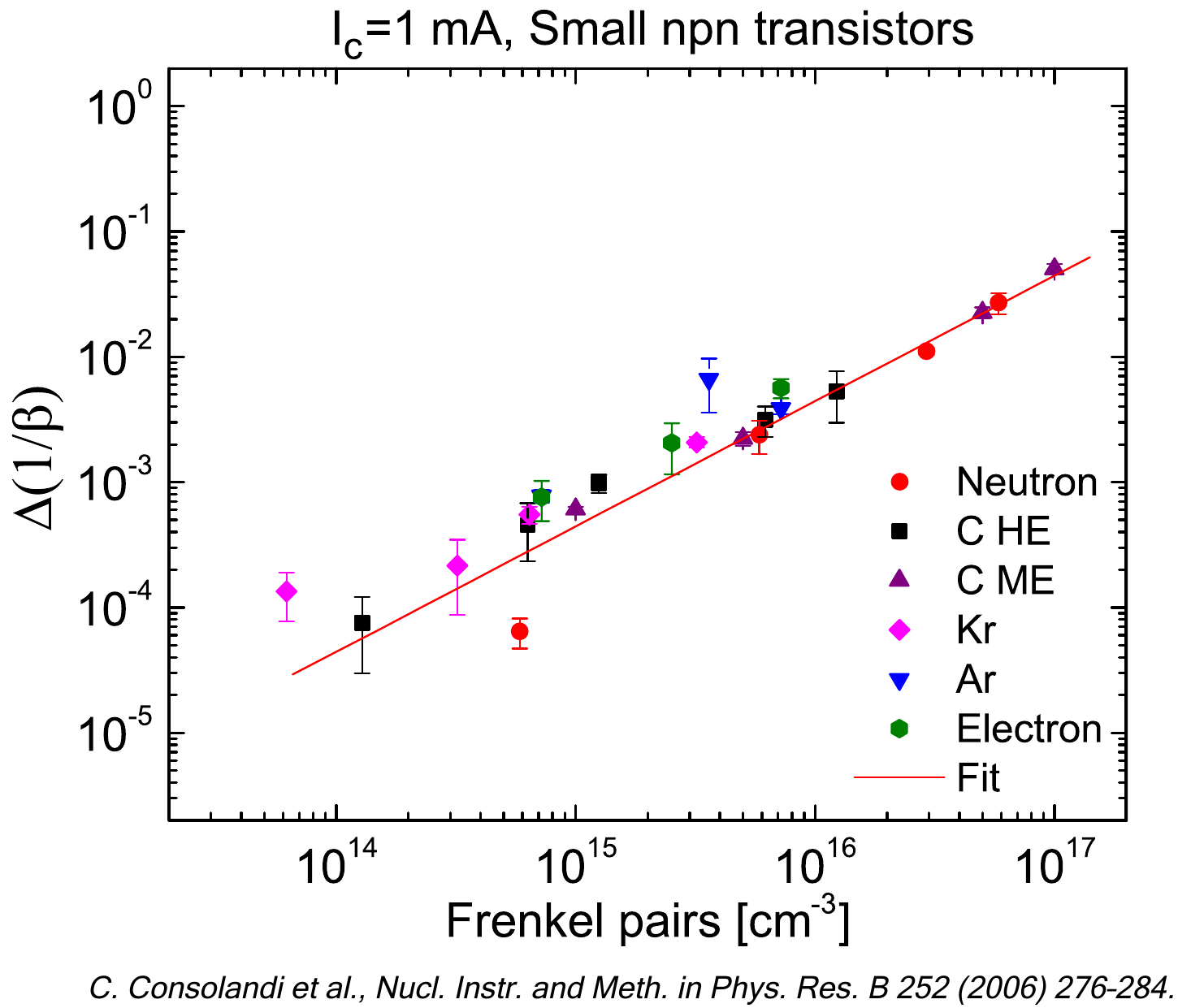SR-NIEL – 7 Screened Relativistic (SR) Treatment for NIEL Dose Nuclear and Electronic Stopping Power Calculator (version 8.0)The present Calculator provides the "probability in cm2 g-1" of energetic recoil nuclei as a function of the incoming particle energy.

### How to use this Recoil Probability Calculator

The Probability for an incoming particle to generate a recoil nuclei due to Coulomb interaction above the requested Eener value is obtained by:

(probability in cm2g-1) x (absorber density in g cm-3) x (traversed thickness in cm),

where the "probability in cm2g-1" is obtained by the web calculator, absorber density and traversed thickness are defined by the user.
Eener is the the lower limit of recoil energy. For information about the computation method one may see the Energetic Nuclear Recoil page.

The recoil energy is deposited by ionizing and non-ionizing processes. For every recoil energy value, the amounts of energy deposited for those processes  are available, for elements, at Energy Partition Function Calculator.

Input parameters and options for the tool are described below. When the input form has been completed, pressing the "CALCULATE" button will start the calculation and open the "Results" page (allow for pop-up in your browser settings). The result page will be also linked at the bottom of the calculator page.

For further information about the computation method are available at the Nuclear Stopping Power for Electrons, Protons and Ions page.

### Input Parameters:

- Incident particle (for Protons&Ions Calculator)
- Electron form (for Electrons Calculator)
- Target material
- Calculator energy limits.

### Incident Particle

In the Protons&Ions Calculator, using the pull down menu, the user can select the species of the incident particle, either a proton or one of the elemental ions.

### Electron Form Factor

In the Electron Calculator, the user can select the nuclear form factor (Exponential, Gaussian or Uniform ) model to be used. The form factor accounts for the spatial distribution of charge density probed in the electron-nucleus scattering.

### Target Material

In this section it is possible to specify the composition of the target material selecting the number of elements in the compound. The required parameters for each element are:

- Atomic number (Z)/Chemical symbol
- Stoichiometric index or element fraction

### Energy Limits

This section define the energy limits of the calculation, and the following parameters will be defined:
- Minimum Energy of the incident particle
- Maximum Energy of the incident particle
- Lower limit of the recoil energy Eener

The output table will contain 20 fixed values for each decade within the above selected range.

### Result

The result page contains the input parameters, the "probability in cm2g-1" curve as a function of the incoming particle energy and the result table.